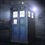# Help needed in NT!

My doubt has been resolved by Satyajit Mohanty and Pi Han Goh.How would you go about solving this problem?:$10^n \equiv 2\pmod{19};n \in \mathbb{Z}^{+}\\ Smallest\ such\ n=?$Please help!5 years, 2 months ago

This discussion board is a place to discuss our Daily Challenges and the math and science related to those challenges. Explanations are more than just a solution — they should explain the steps and thinking strategies that you used to obtain the solution. Comments should further the discussion of math and science.

When posting on Brilliant:

• Use the emojis to react to an explanation, whether you're congratulating a job well done , or just really confused .
• Ask specific questions about the challenge or the steps in somebody's explanation. Well-posed questions can add a lot to the discussion, but posting "I don't understand!" doesn't help anyone.
• Try to contribute something new to the discussion, whether it is an extension, generalization or other idea related to the challenge.

MarkdownAppears as
*italics* or _italics_ italics
**bold** or __bold__ bold
- bulleted- list
• bulleted
• list
1. numbered2. list
1. numbered
2. list
Note: you must add a full line of space before and after lists for them to show up correctly
paragraph 1paragraph 2

paragraph 1

paragraph 2

[example link](https://brilliant.org)example link
> This is a quote
This is a quote
    # I indented these lines
# 4 spaces, and now they show
# up as a code block.

print "hello world"
# I indented these lines
# 4 spaces, and now they show
# up as a code block.

print "hello world"
MathAppears as
Remember to wrap math in $$ ... $$ or $ ... $ to ensure proper formatting.
2 \times 3 $2 \times 3$
2^{34} $2^{34}$
a_{i-1} $a_{i-1}$
\frac{2}{3} $\frac{2}{3}$
\sqrt{2} $\sqrt{2}$
\sum_{i=1}^3 $\sum_{i=1}^3$
\sin \theta $\sin \theta$
\boxed{123} $\boxed{123}$

Sort by:

- 5 years, 2 months ago

The smallest $n$ is $17$. Study residue systems modulo $n$.

- 5 years, 2 months ago

Kindly tell the method!

- 5 years, 2 months ago

Oh,wait I got it:did you do it this way:$10^{18}\equiv1\pmod{19}\\ from\ Euler's\ totient\ function\\ 10^{17}\equiv\dfrac{1}{10}\equiv\dfrac{2}{20}\equiv2\pmod{19}$?

- 5 years, 2 months ago

Yup.

- 5 years, 2 months ago

Ok,thanx!Are you in mood for a trigo problem?

- 5 years, 2 months ago

You have only shown that n = 17 is a solution but you didn't show that it's the smallest solution.

- 5 years, 2 months ago

- 5 years, 2 months ago

Show that the residues of a prime is (prime - 1). So if you found anything less than a prime, then it's minimum.

- 5 years, 2 months ago

You need to check that 17 is the smallest, though.

- 5 years, 2 months ago